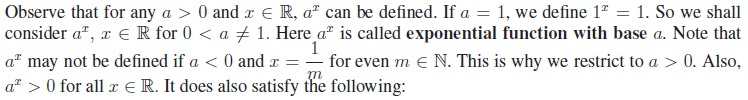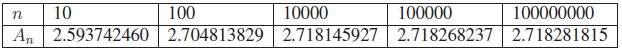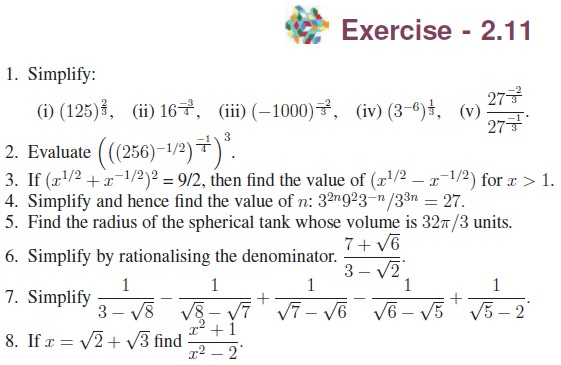Home | | Maths 11th std | Exponents and Radicals

## Chapter: 11th Mathematics : UNIT 2 : Basic Algebra

Mathematics : Basic Algebra

First we shall consider exponents.

## 1. ExponentsQuestion:## 3. Exponential FunctionProperties of Exponential FunctionA Special Exponential Function

Among all exponential functions, f(x) = ex, x R is the most important one as it has applications in many areas like mathematics, science and economics. Then what is this e? The following illustration from compounding interest problem leads to the constant e.

Illustration

Compound InterestWe notice that as n gets really large, An values seem to be getting closer to 2.718281815..... Actually An values approach a real number e, an irrational number. 2.718281815 is an approximation to e. So the compound interest formula becomes A = P ert, where r is the interest rate and P is the principal and t is the number of years. This is called Continuous Compounding.Tags : Definition, Formula, Solved Example Problems, Exercise | Mathematics , 11th Mathematics : UNIT 2 : Basic Algebra
Study Material, Lecturing Notes, Assignment, Reference, Wiki description explanation, brief detail
11th Mathematics : UNIT 2 : Basic Algebra : Exponents and Radicals | Definition, Formula, Solved Example Problems, Exercise | Mathematics